Next: The Hough Transform Up: Computer Vision IT412 Previous: Lecture 6

Subsections

# Classical Feature Detection

It seems clear, both from biological and computational evidence, that some form of data compression occurs at a very early stage in image processing. Moreover, there is much physiological evidence suggesting that one form of this compression involves finding edges and other information-high features in images. Edges often occur at points where there is a large variation in the luminance values in an image, and consequently they often indicate the edges, or occluding boundaries, of the objects in a scene. However, large luminance changes can also correspond to surface markings on objects. Points of tangent discontinuity in the luminance signal (rather than simple discontinuity) can also signal an object boundary in the scene.

So the first problem encountered with modeling this biological process is that of defining, precisely, what an edge might be. The usual approach is to simply define edges as step discontinuities in the image signal. The method of localising these discontinuities often then becomes one of finding local maxima in the derivative of the signal, or zero-crossings in the second derivative of the signal. This idea was first suggested to the AI community, both biologically and computationally, by Marr , and later developed by Marr and Hildreth , Canny [1,2], and many others [3,4].

In computer vision, edge detection is traditionally implemented by convolving the signal with some form of linear filter, usually a filter that approximates a first or second derivative operator. An odd symmetric filter will approximate a first derivative, and peaks in the convolution output will correspond to edges (luminance discontinuities) in the image. An even symmetric filter will approximate a second derivative operator. Zero-crossings in the output of convolution with an even symmetric filter will correspond to edges; maxima in the output of this operator will correspond to tangent discontinuities, often referred to as bars, or lines.

In this lecture we will begin by considering the classical approaches to detecting edges in images: first derivative techniques, second derivative techniques, and surface fitting. We will then consider how features other than edges might be detected.

## First order differential methods of edge detection

Most edge detection methods work on the assumption that an edge occurs where there is a discontinuity in the intensity function or a very steep intensity gradient in the image. Using this assumption, if we take the derivative of the intensity values across the image and find points where the derivative is a maximum, we will have marked our edges.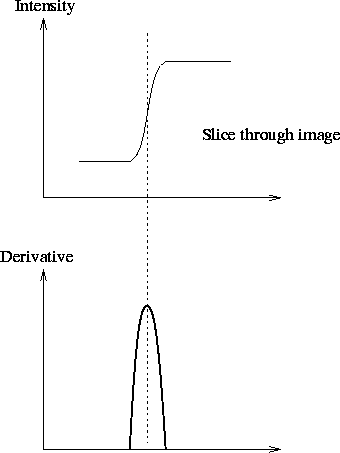In a discrete image of pixels we can calculate the gradient by simply taking the difference of grey values between adjacent pixels.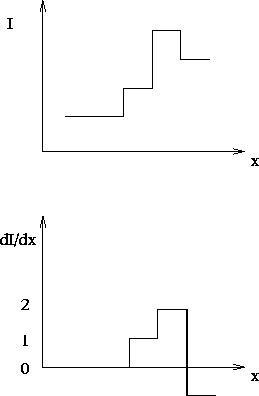This is equivalent to convolving the image with the mask [-1,1]. Note that we have a problem in determining where we place the result of the convolution. Ideally we would like to place it between the two pixels. By using a mask that spans an odd number of pixels we end up with a middle pixel to which we can assign the convolution result.

The gradient of the image function I is given by the vector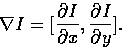The magnitude of this gradient is given by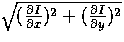and its direction by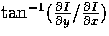. Note, one can use any pair of orthogonal directions to compute this gradient, although it is common to use the x and y directions.

The simplest gradient operator is the Robert's Cross operator and it uses the masks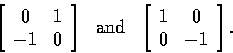Thus the Robert's Cross operator uses the diagonal directions to calculate the gradient vector.

A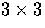approximation to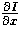is given by the convolution mask

 -1 0 1 -1 0 1 -1 0 1
This definesfor the Prewitt operator, and it detects vertical edges. The Sobel operator is a variation on this theme giving more emphasis to the centre cell. The Sobel approximation tois given by
 -1 0 1 -2 0 2 -1 0 1
Similar masks are constructed to approximate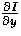,thus detecting the horizontal component of any edges. Both the Prewitt and Sobel edge detection algorithms convolve with masks to detect both the horizontal and vertical edge components; the resulting outputs are simply added to give a gradient map. The magnitude of the gradient map is calculated and then input to a routine that suppresses (to zero) all but the local maxima. This is known as non-maxima suppression. The resulting map of local maxima is thresholded (small local maxima will result from noise in the signal) to produce the final edge map. It is the non-maxima suppression and thresholding that introduce non-linearities into this edge detection scheme. Moreover, the output of the thresholding stage is extremely sensitive and there are no automatic procedures for satisfactorily determining thresholds that work for all images. This is evident from the edge maps of the mandrill image shown below.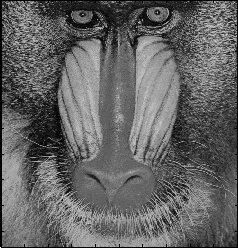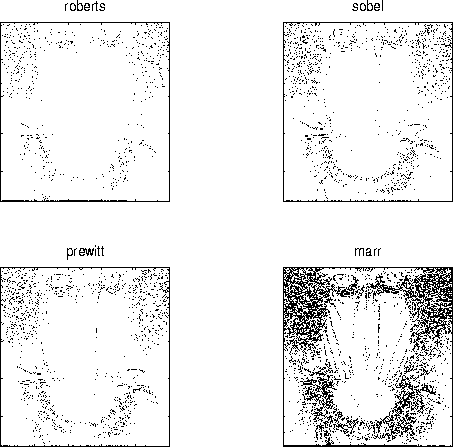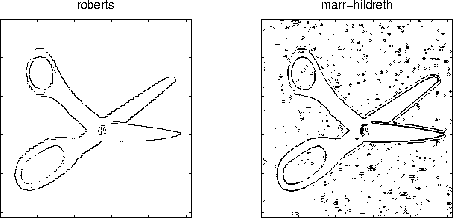The main problem one has to deal with in differential edge detection schemes is noise. The spikes in the derivative from the noise can mask the real maxima that indicate edges. If we smooth the image then the effects of noise can be reduced.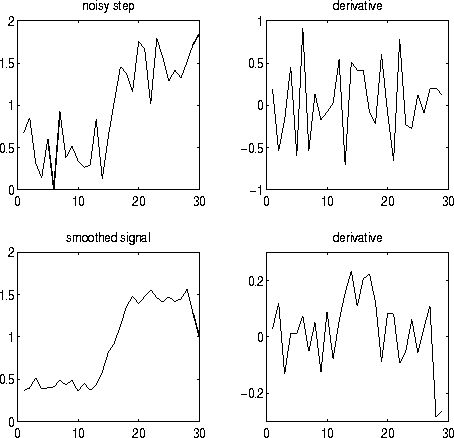A procedure we could use is
1.
Convolve the image with a Gaussian mask to smooth it, then
2.
calculate derivatives of the smoothed image.
Both these operations are linear, and we can combine them in a different, more computationally efficient way. If we calculate the derivative of the Gaussian mask and convolve the image with this mask we compute the derivative of the smoothed image in one hit. That is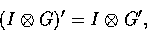where I is the image and G is the Gaussian mask.

## Second order derivative operators

A maximum of the first derivative will occur at a zero crossing of the second derivative.

To get both horizontal and vertical edges we look at second derivatives in both the x and y directions. This is the Laplacian of I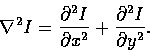The Laplacian is linear and rotationally symmetric.

Thus, we search for the zero crossings of the image that is first smoothed with a Gaussian mask and then the second derivative is calculated; or we can convolve the image with the Laplacian of the Gaussian, also known as the LoG operator;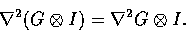This defines the Marr-Hildreth operator.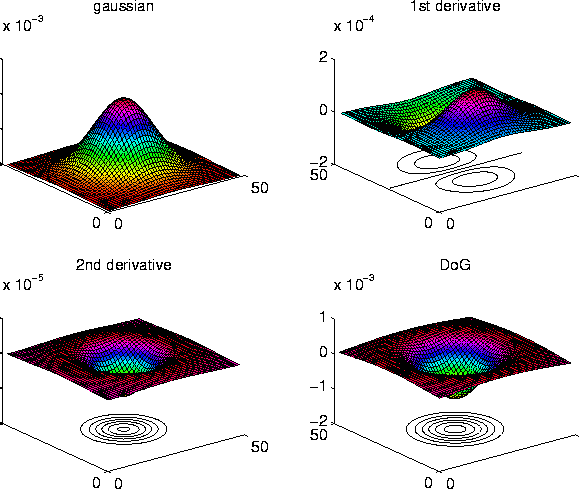One can also get a shape similar to G'' by taking the difference of two Gaussians having different standard deviations. A ratio of standard deviations of 1:1.6 will give a close approximation to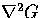.This is known as the DoG operator (Difference of Gaussians), or the Mexican Hat Operator.

The Marr-Hildreth operator became widely used for the following reasons:

1.
2.
Researchers found receptive fields in the eyes of animals (usually cats and macaque monkeys) that behaved just like this operator.
3.
The operator is symmetric. Edges are found in all orientations, unlike the first derivative operators which are directional.
4.
Zero crossings of the second derivative are simpler to determine than are maxima in the first derivative; all that needs to be done is to look for a sign change in the signal. Moreover, the zero crossings of a signal always form closed contours. This is nice if one is trying to separate out objects in the scene.

There are some problems, however.

1.
Being a second derivative operator the influence of noise is considerable.
2.
Animals have lots of other types of receptive fields so this is not the whole story.
3.
Always generating closed contours is not realistic.
4.
The Marr-Hildreth operator will mark edges at some locations that are not edges.

## The Canny edge detector

The current standard edge detection scheme widely used around the world is the Canny edge detector. This is the work John Canny did for his Masters degree at MIT in 1983. He treated edge detection as a signal processing problem and aimed to design the optimal' edge detector. He formally specified an objective function to be optimised and used this to design the operator.

The objective function was designed to achieve the following optimisation constraints:

1.
Maximize the signal to noise ratio to give good detection. This favours the marking of true positives.
2.
Achieve good localization to accurately mark edges.
3.
Minimize the number of responses to a single edge. This favours the identification of true negatives, that is, non-edges are not marked.

Note a difference-of-boxes operator will maximize the signal to noise ratio, but will give several responses to a single edge.

After some complicated analysis Canny came up with a function that was the sum of 4 exponential terms. However, this function looked very much like a first derivative of a Gaussian! So this is what ended up being used.

The overall edge detection procedure he developed was as follows:

1.
We want to find the maxima of the partial derivative of the image function I in the direction orthogonal to the edge direction, and to smooth the signal along the edge direction. Thus Canny's operator looks for the maxima of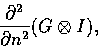where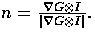However, many implementations of the Canny edge detector actually approximate this process by first convolving the image with a Gaussian to smooth the signal, and then looking for maxima in the first partial derivatives of the resulting signal (using masks similar to the Sobel masks). Thus we can convolve the image with 4 masks, looking for horizontal, vertical and diagonal edges. The direction producing the largest result at each pixel point is marked. Record the convolution result and the direction of the edge at each pixel.
2.
Perform non-maximal suppression. Any gradient value that is not a local peak is set to zero. The edge direction is used in this process.
3.
Find connected sets of edge points and form into lists.
4.
Threshold these edges to eliminate insignificant' edges. Canny introduced the idea of thresholding hysteresis. This involves having two different threshold values, usually the higher threshold being 3 times the lower. Any pixel in an edge list that has a gradient greater than the higher threshold value are classed as a valid edge point. Any pixels connected to these valid edge points that have a gradient value above the lower threshold value are also classed as edge points. That is, once you have started an edge you don't stop it until the gradient on the edge has dropped considerably.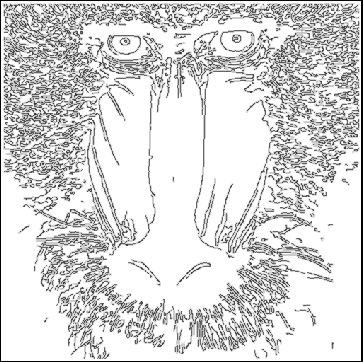## Problems with gradient-based edge detectors

Gradient-based edge detection schemes suffer from a number of problems, but they are still the most commonly used by the computer vision community. Some of these problems include the following:
1.
You have to choose threshold values and the width of your masks. This problem is common to all gradient based methods. Note that if you double the size of an image leaving its grey values unchanged all the gradients will be halved. This complicates the setting of any threshold. An additional problem is that the width of the mask (and hence the degree of smoothing) affects the positions of zero crossings and maximum intensity gradients in the image. The estimated position of any edge should not be affected by the size of the convolution mask.
2.
Corners are often missed because the 1D gradient at corners is usually small. This can cause considerable difficulties for line labeling schemes because they rely on having corners and junctions being marked properly.
3.
First derivative operators will only find step-like features. If you want to find lines you need a different operator. For example, to find bar features you could look for peaks (not zero crossings) from a second derivative operator. Canny did design an operator for finding lines.
Thus, differential edge detection schemes suffer both from false positives and false negatives.

## Surface fitting

An alternative approach to edge detection is to develop an edge model and then to compute its degree of match to the image data. In general, though, surface fitting is computationally more expensive than differential approaches to edge detection.

An edge is modeled by specifying its four degrees of freedom: its position, its orientation, and the constant intensities on either side of the step. We could simply match the data by seeking the least squares error fit of the parametric model to the image window, but such an approach is generally computationally very expensive. Normally what is done is that both the image data and the model are represented over small windows by their first 8 coefficients in a particular two-dimensional orthonormal series expansion. In this case the optimisation reduces to just one variable: the orientation of the edge. An edge is declared present when the weighted least squares error is smaller than some pre-set threshold.

## Edge detector performance

A number of researchers have considered the problem of measuring edge detector performance. In fact, it is difficult since we don't really know what the underlying features are that we wish to detect. However, if we assume that they are step edges corrupted by Gaussian noise, then some criteria can be set for evaluating performance. Such criteria are usually the following:

• the probability of false edges;
• the probability of missing edges;
• the error in estimating the edge angle;
• the mean square distance of the edge estimate from the true edge; and
• the algorithm's tolerance to distorted edges and other features such as corners and junctions.

The first two criteria relate to edge detection, the second two to edge localisation, and the last to tolerance to departures from the ideal edge model. Pratt  introduce\$ function FM for measuring quantitatively the performance of various edge detectors. His measure is

FM = 1
 max (IA,II)
IA
å
i = 1

1
1 + di a2
,
where IA, II, d, and a are respectively the detected edges, the ideal edges, the distance between the actual and the ideal edges, and a design constant used to penalise displaced edges.Next: The Hough Transform Up: Computer Vision IT412 Previous: Lecture 6
Robyn Owens
10/29/1997